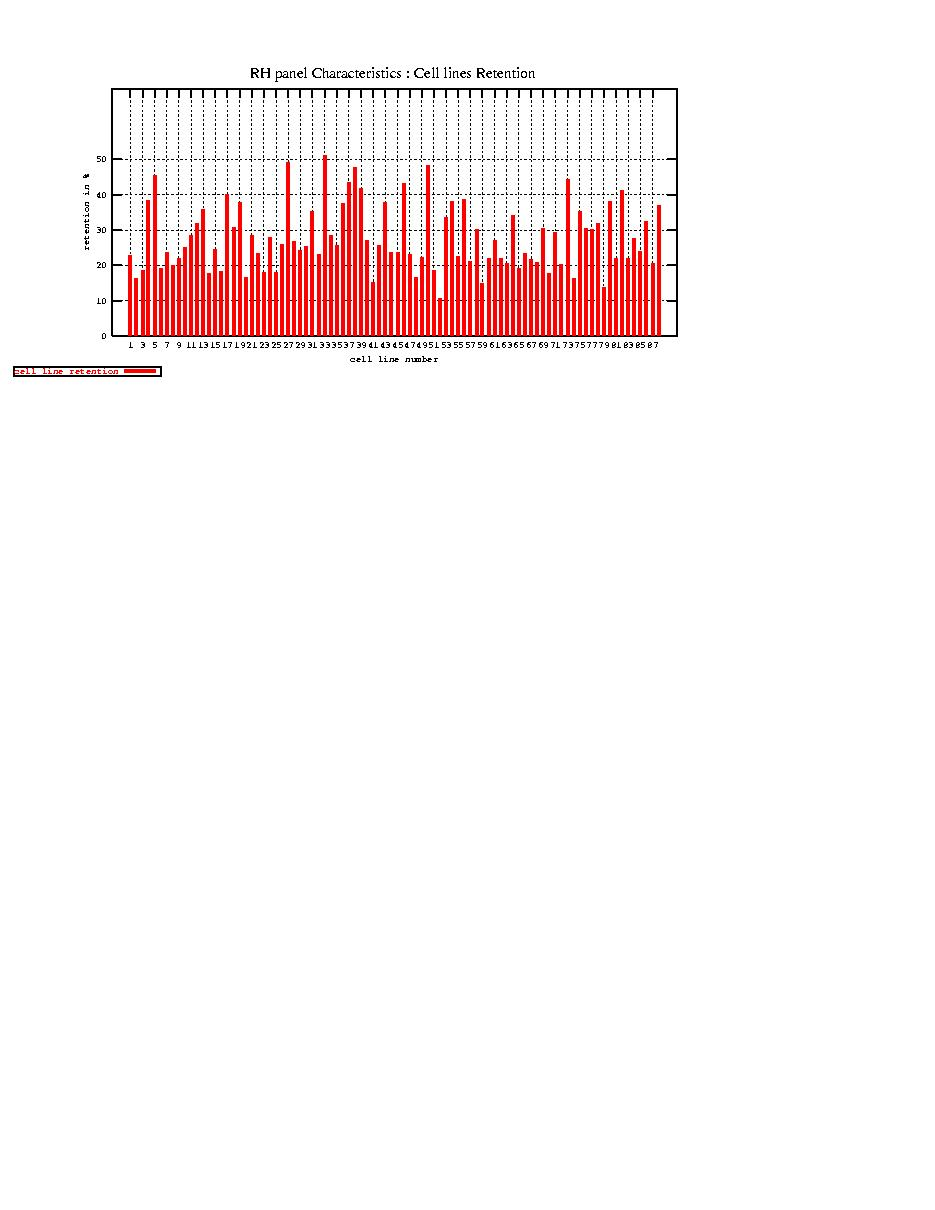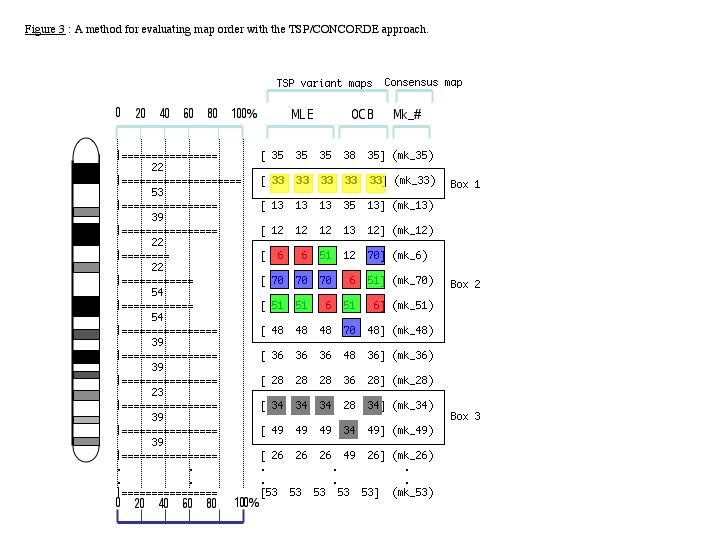```
============================================================================
SOM for RH Panel construction

A new dog/hamster radiation hybrid panel with a higher resolution limit
has been constructed by fusing dog primary fibroblasts irradiated at
9000 rads with thymidine kinase-deficient hamster cells HTK3 :

I ) Cells:  Dog primary fibroblasts were derived from two male mongrel
dogs.  Hamster HTK3- cells (thymidine kinase defficient) were derived
from A2H strain after 6BrdU selection.

with 9000 rad.  B) Chemically induced fusion was done using
PEG2000+10% DMSO with 20X106 HTK3-cells (Dog/Hamster cell ratio = 1.0)

III ) Results: Five identical fusions were done which enabled us to
produce 400 clones. Average fusion efficiency was 4 per 106 (4 clones
for every 106 dog fibroblasts).  The 400 clones have been tested for
their retention frequency using a set of 96 defined BAC markers.  88
clones were selected and expanded, resulting in a panel with an average
retention frequency of 29% and a resolution of 300 kb.

- Cell lines retention
(percentage are derived from 10,500 RH genotyping data).
Cell Line n.1 = 23.0071 %
Cell Line n.2 = 16.3397 %
Cell Line n.3 = 18.6298 %
Cell Line n.4 = 38.3902 %
Cell Line n.5 = 45.3957 %
Cell Line n.6 = 19.1613 %
Cell Line n.7 = 23.8477 %
Cell Line n.8 = 20.0019 %
Cell Line n.9 = 22.0118 %
Cell Line n.10 = 25.2005 %
Cell Line n.11 = 28.4858 %
Cell Line n.12 = 32.0224 %
Cell Line n.13 = 35.7909 %
Cell Line n.14 = 17.7215 %
Cell Line n.15 = 24.5531 %
Cell Line n.16 = 18.4559 %
Cell Line n.17 = 40.0425 %
Cell Line n.18 = 30.7952 %
Cell Line n.19 = 37.8104 %
Cell Line n.20 = 16.7552 %
Cell Line n.21 = 28.4279 %
Cell Line n.22 = 23.5578 %
Cell Line n.23 = 18.1177 %
Cell Line n.24 = 27.8771 %
Cell Line n.25 = 18.0501 %
Cell Line n.26 = 26.0798 %
Cell Line n.27 = 49.3091 %
Cell Line n.28 = 26.7949 %
Cell Line n.29 = 24.2246 %
Cell Line n.30 = 25.4517 %
Cell Line n.31 = 35.4334 %
Cell Line n.32 = 23.2969 %
Cell Line n.33 = 51.0387 %
Cell Line n.34 = 28.5148 %
Cell Line n.35 = 25.674 %
Cell Line n.36 = 37.5882 %
Cell Line n.37 = 43.5791 %
Cell Line n.38 = 47.8404 %
Cell Line n.39 = 41.8688 %
Cell Line n.40 = 27.0268 %
Cell Line n.41 = 15.3445 %
Cell Line n.42 = 25.7996 %
Cell Line n.43 = 37.965 %
Cell Line n.44 = 23.5965 %
Cell Line n.45 = 23.7318 %
Cell Line n.46 = 43.2022 %
Cell Line n.47 = 23.21 %
Cell Line n.48 = 16.533 %
Cell Line n.49 = 22.2534 %
Cell Line n.50 = 48.2655 %
Cell Line n.51 = 18.5718 %
Cell Line n.52 = 10.5904 %
Cell Line n.53 = 33.5395 %
Cell Line n.54 = 38.1486 %
Cell Line n.55 = 22.5722 %
Cell Line n.56 = 38.738 %
Cell Line n.57 = 21.1711 %
Cell Line n.58 = 30.1092 %
Cell Line n.59 = 15.0449 %
Cell Line n.60 = 21.9152 %
Cell Line n.61 = 27.1041 %
Cell Line n.62 = 22.0118 %
Cell Line n.63 = 20.6687 %
Cell Line n.64 = 34.0613 %
Cell Line n.65 = 19.1323 %
Cell Line n.66 = 23.3936 %
Cell Line n.67 = 21.7992 %
Cell Line n.68 = 20.8909 %
Cell Line n.69 = 30.5827 %
Cell Line n.70 = 17.7215 %
Cell Line n.71 = 29.3458 %
Cell Line n.72 = 20.3015 %
Cell Line n.73 = 44.4487 %
Cell Line n.74 = 16.2335 %
Cell Line n.75 = 35.2401 %
Cell Line n.76 = 30.3701 %
Cell Line n.77 = 30.1962 %
Cell Line n.78 = 32.0128 %
Cell Line n.79 = 13.9434 %
Cell Line n.80 = 38.2452 %
Cell Line n.81 = 21.9152 %
Cell Line n.82 = 41.2407 %
Cell Line n.83 = 22.1084 %
Cell Line n.84 = 27.7708 %
Cell Line n.85 = 23.9443 %
Cell Line n.86 = 32.3799 %
Cell Line n.87 = 20.6977 %
Cell Line n.88 = 37.0374 %

RH panel average retention : 29 %

Histogram of cell line retention [-full size-]====================================================================================
SOM for Markers identification

All canine sequence were identified through BLASTn best mutual
alignement with the human genome.Human genes that had a representative
dog sequence were allotted to 11818 bins . The human genome was split
into 75 kb segments and the sequence providing the highest blastn score
in each of the 11818 binsAlignment was retained whenthe dog/human
alignment overlaps at least one human exon defined by the 24,567 genes
of Ensembl version 13.31.1

All selected dog sequences are contigs or singletons, derived from the
assembly of 6.22 million reads. All dog sequence were searched against
the human genome (NCBI 31) by blastn.

Selection criteria :

1. Blastn alignment with human genome yields mutually-best HSPs.

HSPs are defined as mutually-best when the segment of
aligned dog sequence has no higher-scoring hit elsewhere on the human
genome AND the segment of aligned human sequence has no higher-scoring
alignment with another dog sequence.

2. The dog/human alignment overlaps at least one human exon

Defined by the 24,567 genes of Ensembl version 13.31.1

3. The dog sequence is not a processed pseudogene

Where multiple HSPs were separated by <25 bases on a dog
sequence, but >300 bases on the human sequence, the dog sequence was
considered as a potential pseudogene, and was eliminated.

4. The dog/human alignment includes only part of the dog sequence

A dog sequence for which the alignment extended over the
complete length of the dog sequence may not yield dog-specific primers,
and was eliminated.

5. After applying criteria 1-4, selection of a single dog sequence for
each human gene    The sequence providing the highest blastn score.

6. Human genes that have a representative dog sequence were allotted to
11818 bins

i.e. The human genome was split into 75 kb segments. If
multiple human genes fell within a single segment, these were binned
together.

7. Selection of a single dog sequence for each 75 kb bin

The sequence providing the highest blastn score in each of
the 11818 bins.

8. Selection of a dog sequence from alternate bins

This gave 5909 sequences for the first round of mapping.
There remains another 5909 dog sequences from the remaining 75 kb bins
that can be used for the second round.

================================================================================
SOM for Map construction

Map construction was carried out with the rh_tsp_map2 package (Agarwala
et al. 2001) that uses the well-known state of art CONCORDE algorithm
in the TSP problem (Applegate et al.) The RH vectors were analysed by
pairwise analysis using the pairlods_dists option to constitute linkage
groups. The Traveling Salesman Problem (TSP) method using the CONCORDE
chained Lin-Kernighan algorithm of the rh_tsp_map2 package was used to
order of each linkage group. Parametric and non-parametric analysis is
systematically provided resulting in 5 independent maps. A consensus
map could be derived from the 5 independent maps along with a mapping
support expressed in percentage assigned to each marker.Legend :

The rh_tsp_map2 program delivers 5 maps.
Results of the comparison of these five maps
is enlighted by horizontal bars.
When the same marker is present in the
five maps at a given position the
horizontal bars have a maximum length
and correspond to mapping positions
reaching high confidence ( i.e. box 1).
Map positions occupied by two or more
markers are characterized by shorter horizontal
bars (i.e. boxes 2 and 3).
Although as in box 2 a given marker can
occupied three different positions
extending the size of the scrambled
region, in box 3 only two adjacent
markers exchange their positions
limiting the zone of uncertainty

from Hitte et al. 2003

================================================================================
SOM : MAP RESOURCES

Unix users :
2: gunzip ALL-seq.tar.gz # uncompress the file
3: tar xf ALL-seq.tar    # each chromosome are distributed in individual tar ball.
4: tar xf CFAxx.tar      # uncompress individual chromosome files

The High resolutive canine RH panel is available upon request :  [- ask for RH panel -]

```